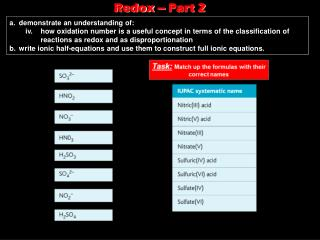DownloadDownload PresentationRedox – Part 2

Redox – Part 2

Download PresentationRedox – Part 2

- - - - - - - - - - - - - - - - - - - - - - - - - - - E N D - - - - - - - - - - - - - - - - - - - - - - - - - - -
Presentation Transcript

1. a. demonstrate an understanding of: • iv. how oxidation number is a useful concept in terms of the classification of reactions as redox and as disproportionation • b. write ionic half-equations and use them to construct full ionic equations. Redox – Part 2

2. Task:Match up the formulas with their correct names

3. Naming Compounds • Some elements can have more than one oxidation number. • So to avoid confusion when naming the compounds they form, its helpful to use these numbers in their names.

4. What are the missing oxidation numbers here for Manganese and Chromium, respectively? Naming Compounds ? ?

6. Redox and Oxidation Numbers • An increase in oxidation number means oxidation has occurred. • While a decrease in oxidation number means reduction has occurred. • E.g. What would be the oxidation numbers for each of the species in this reaction? +1 -2 +1 -1 0 0 Therefore, chlorine has been reduced, and sulphur has been oxidised.

7. Redox and Oxidation Numbers • Another example is the Thermite Reaction (click here to see it in action!!) • But what is oxidised and what is reduced? 0 -2 +3 -2 0 +3 Therefore, iron has been reduced, and aluminium has been oxidised.

8. Disproportionation • Is a type of reaction in which a substances both oxidised and reduced in the same reaction. E.g. the breakdown of hydrogen peroxide:

9. Disproportionation – another example • The reaction of copper(I) oxide with dilute sulphuric acid: • Study the equation carefully – what disproportionates? • The answer is copper (Cu) which gets oxidised from +1 to +2 and also reduced from +1 to zero, in the same reaction. • Other examples of such reactions include reactions of chlorine with water, and chlorine with hot sodium hydroxide solution.

10. Questions

12. Oxidation States • Some elements show a range of oxidation states. • One colourful example is the reduction of vanadium (V) to vanadium (II) through sucessive oxidation number.

13. Vanadium – its different oxidation states Ammonium Vanadate (V) (white solid) can be added to dilute HCl to give the orange Dioxovanadium (V) ion: VO3- + 2H+ VO2+ + H2O +5 +5 If granulated zinc is now added it reduces the vanadium over a period of several minutes and gives several colour changes: Blue [VO(H2O)5]2+ Vanadium (IV) Green [VCl2(H2O)4] + Vanadium (III) Violet [V(H2O)6]2+ Vanadium (II)

14. Common Oxidising Agents • What is an oxidising agent? • A species that reacts by oxidising something else and getting reduced itself. • So, as we will see, electrons in the half equations are always on the left-hand side of the equation, as oxidising agents gain electrons.

15. More Oxidising Agents

16. More Oxidising Agents

17. Common Reducing Agents • What is an reducing agent? • A species that reacts by reducing something else and getting oxidised itself. • So, this time, electrons in the half equations are always on the right-hand side of the equation, as reducing agents loose electrons.

18. More Reducing Agents

19. More Reducing Agents

20. Firstly, write down the half equations you need. Writing balanced equations from ionic half equations - Worked Example…

21. Worked Example…..cont…. Notice, that in adding the two equations together The electrons are cancelled out and not Included in the final equation

22. Again, start with the half equations Worked Example 2… Have a go before you turn to the next slide for the answer